# Arithmetic - math word problems

#### Number of problems found: 2620

• Compare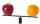Compare with characters >, <, =: 85.57 ? 80.83
• Cubic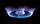Convert 660 m3 of natural gas volume to energy units kWh. Combustion of 1 cubic meter of natural gas is released energy 10.55 kWh.
• AverageThe arithmetic mean of the two numbers is 71.7. One number is 5. Calculate the second number.
• Gauss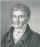Help little C.F. Gauss sum all the integers from 1 to 400.
• TriangleProve whether you can construct a triangle ABC, if a=9 cm, b=6 cm, c=10 cm.
• School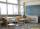27 students of 6.D class are going on a trip. They pay 9 € each. The teacher got 153 €. How many children have not paid?
• ProductThe product of two consecutive odd numbers is 8463. What are this numbers?
• Angle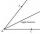Draw angle |∠ ABC| = 130° and built its axis. What angle is between axis angle and arm of angle?
• Circles 2Calculate the area bounded by the circumscribed and inscribed circle in triangle with sides 12 cm, 14 cm, 18 cm.
• Money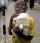Everyone complains about the lack of money. In fact, there is no shortage of money, but the relative scarcity of goods and things that people buy for the money. Calculate what percentage of the price of all goods and services rose immediately, if everyone
• SphereThe surface of the sphere is 12100 cm2, and the weight is 136 kg. What is its density?
• Shots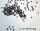5500 lead shots with diameter 4 mm are decanted into a ball. What is its diameter?
• Subsets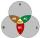How many are all subsets of set C = (97, 67, 66, 18, 59, 64)?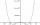By introducing a new variable solve biquadratic equation: - x 4 +277 x2 -15876=0
• Powers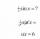Is true for any number a,b,c,d equality:? ?
• Is equal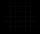Is equal following terms? -9 21 = (-9) 21
• Binomials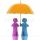To binomial 36x2-168x add a number to the resulting trinomial be square of binomial.
• Root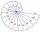Use law of square roots roots: ?
• Rates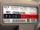When gas consumption, the consumer may choose one of two rates: rate A - which pays 0.4 € per 1 m3 of gas a flat monthly fee of 3.9 € (regardless of consumption) rate B - which pays 0.359 € per 1 m3 of gas a flat monthly fee of 12.5 € From what monthlyDetermine the numbers b, c that the numbers x1 = -1 and x2 = 3 were roots of quadratic equation: -3x 2 + b x + c = 0